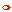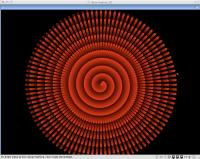(anonymous IP: 3.235.188.113,297)

 CategoriesAudio (92)Datatype (15)Demo (41)Development (239)Document (55)Driver (18)Emulation (59)Game (503)Graphics (207)Library (22)Network (57)Office (20)Utility (244)Video (18)

Total files: 1590

Full index file
Recent index file

Part of aros exec
 Readme for:  Development » Language » sdl_basic-1.0.2.i386-aros.tar.gz

# Sdl_basic-1.0.2.i386-aros

Description: This is an implementation of the language BASIC
Size: 288kb
Version: 1.0.2
Date: 02 Oct 09
Author: Dave Ashley
Submitter: Miloslav Martinka
Homepage: http://www.linuxmotors.com/
Requirements: i386 AROS
Category: development/language
Distribute: yes
FileID: 615

 Snapshots:Snapshots: 1
Videos: 0
244  (Accumulated)
Votes: 0 (0/0)  (30 days/7 days)

[Show comments] [Show snapshots] [Show videos] [Show content] [Replace file]
```This is an implementation of the language BASIC I made to expose my kids
to programming. You should be able to build under linux if you have SDL
installed, just do

make
./basic

There are a number of sample basic programs included. Within basic just
do

run

or
run

etc.
cool9 is an "asteroids" game.

Arrow keys work for moving within the typed line, and through a line history.
The page up and down keys, or shifted page up and down keys, scroll through
a text history.

**** BUILDING ON MAC OSX:
If the SDL framework is installed in /Library/Frameworks/SDL.framework
you should be able to build by just doing
make -f Makefile.osx

Basic interpreter commands. Commands can be shortened

edit #             = edit line, arrow keys, delete, backspace, always in
insert mode
ren [#[,#]]          = renumber lines, first number is step count, 2nd start #
save filename      = save out program to file (appends .bas automatically)
run                = Initialize everything run program
exit               = Exit the program
<statement>        = Can execute any statement
list [#[-#]]       = list program or parts of it
new                = Delete program + all variables
scr                = same as new
info               = Print out info on current program
parse              = Parse the basic program and dump the virtual machine code
help  --- prints out help, more detail than this file

Multiple statements can be on a line, separated by ':'.

Variables are any string starting with a-z. Case is not sensitive. Can
contain numbers. Up to 16 characters. Might not be able to start with a
keyword.

[let] variable = expression
goto #
gosub #
return
on expr goto #, ...          ;one based
rem <comment>
dim variable(#[,...])  ; arrays are one based
for variable = expression to espression [step expression]
next [variable]
print string|expression[,|;][...][,|;]
color <r>,<g>,<b>[,<alpha>]   ; range 0-255 each
cls  ; clear screen to black
fill ; fill screen with current pen color
pen <diameter> ; always circular for now, defaults to '1'
move <x>,<y>
spot
line <x>,<y>
NOT IMPLEMENTED:flood <x>,<y> ; does flood fill
NOT IMPLEMENTED: curve <cx>,<cy>,<x>,<y>  ; bezier curve
rectangle <x1>,<y1>,<width>,<height>
box <x1>,<y1>,<1/2 width>,<1/2 height>
rect <x1>,<y1>,<1/2 width>,<1/2 height>
NOT IMPLEMENTED:text <x1>,<y1>,<string>
NOT IMPLEMENTED:randomize
input variable|string
sleep <time>  ; in seconds
if ifexpr then statement|#
data expression[,...]
end
if <condition> then statements
if <condition> then statements else statements
tone #, freq #, dur #, wsqr|wsin|wsaw|wtri, fmul #, vol #
See the "help tone" within basic itself...
quiet [voice #]

Functions:

int(expr) = round down to next lowest integer. int(-.5) = -1
fix(expr) = round to integer closest to 0. fix(-.5) is 0
abs(expr) = absolute value
sgn(expr) = sgn(0) is 0. sgn(5) is 1. sgn(-10) is -1.
sqr(expr) = square root of the expr. sqr(9) is 3
sin(expr) = sine
cos(expr) = cosine
log(expr) = log base e
exp(expr) = e raised to the power of the expr
pow(expr,expr)  (pow(x,y) = x raised to the power of y. pow(2,3) is 8
rnd(expr)  (if expr<>0, random number between 1 and expr) if expr=0,
random number between 0 and 1.
atn(expr) = atan -- inverse tangent
atn2(expr1, expr2) = atan2 -- expr1 is the Y and expr2 is the X
tan(expr) = sin(expr)/cos(expr)
sleep(expr) = sleep for some number of seconds -- returns actual time slept
key(expr) = 1 if keyboard key is pressed, 0 otherwise
len(string_expr) = length of the string
val(string_expr) = decimal value of the string. val("1.23") is 1.23
asc(string_expr) = ascii value of first character of string
note(expr) = Frequency in HZ of midi note number of expr. note(69) is 440.0
Useful for

Expression operators, from lowest priority to highest
OR           As in if j=1 or k=2 ...
AND          As in if j=1 and k=2 ...
=, <>, <, >, <=, >=    Comparisons
|, ^         Logical or and xor
&            Logical and
<<, >>       Logical left and right shift
+, -         Plus, minus
*, /         Multiply, divide
~            Power -- 2~3 is 8

(expr)
func(expr[,...])

String expressions:
variable\$
variable\$ + variable\$
mid\$(variable\$, start, length) = interior part of string.
mid\$("abcdef", 3, 2) is "cd"
left\$(variable\$, length) = left part of string.
left\$("abcdef", 3) is "abc"
right\$(variable\$, length)
right\$(abcdef", 3) is "def"
chr\$(expr) = Make a string with one character using the ascii code of the expr.
chr\$(48) is "0"
str\$(expr) = Make a string containing the ascii representation of the expr.
str\$(1.23) is "1.23"
string\$(expr, string_expr) = Make a string using the first character of the
string expression argument, of length of the first expression.
string\$(8, "x") is "xxxxxxxx"

SPECIAL variables

mousex = mouse x position
mousey = mouse y position
mouseb = state of mouse buttons
xsize = x size in pixels of BASIC window
ysize = y size in pixels of BASIC window
ticks = # of ticks (thousdandths of a second) since the program started
keycode = keycode of next key pressed, or -1. Figure out the codes on your own:
10 k=keycode
20 if k=-1 then 10
30 print k
40 goto 10

inkey\$ = ascii code of next key pressed, or an empty string. Not as useful
as keycode since you only get 8 bits...

---------------------------------------------------------------------

THEORY

SDL_basic is divided into these pieces

main.c      =   opens the SDL library and initializes a basic context
basic.c     =   interprets commands
grammar.y   =   a bison grammar and tokenizer for BASIC.
vmachine.c  =   a virtual stack based machine, similiar to FORTH
vdis.c      =   a disassembler for the virtual machine code
misc.h      =   catch all header file
keyboard.c  =   Deals with user input
font.c      =   Deals with text rendering and the scrollback feature
sound.c     =   Handles the sound system
ftgrays.c   =   Rasterizing engine from the freetype library. Used for graphics.
render.c    =   All the graphics rendering functions

When you type "run" the bison parser is invoked. It parses the program
and converts it to virtual machine instructions. These are then executed
by the virtual machine.

In order to add a new command to the language, you need to do these steps:

1) Add appropriate grammar to grammar.y, plus code to emit the correct
virtual machine instructions (steps)
1a) That might require adding a new TOKEN up at the top part of grammar.y
1b) Plus add to the yylex function the ability to recognize the new token.
2) Add to the vdis.c so the new code(s) can be disassembled correctly
3) Add to misc.h any new functions in vmachine.c that need to be added
4) Add new functions to vmachine.c
5) Add to help.c describing the new command(s)

-------------------------------------------
Compiling for AROS, Miloslav Martinka.
-------------------------------------------

```